# NPS calculator: Know how to calculate your National Pension Scheme money

72
In this article, we explain how to use the NPS calculator, to know the pension and lump-sum amount that an NPS subscriber can expect, on maturity or 60 years of age.
The NPS calculator allows one to know the amount of pension per month under the government’s National Pension Scheme, rechristened as the National Pension System or the NPS scheme.
The NPS calculator illustrates the tentative pension and lump-sum amount an NPS subscriber can expect at 60 years of age or on maturity, based on one’s regular monthly contributions, percentage of the corpus that is reinvested for purchasing an annuity and assumed rates in respect of returns on investment and annuity.

## NPS calculator: Step-wise process to use it online

Step 1: Visit the National Pension System Trust’s (NPS Trust) official website at www.npstrust.org.in.Step 2: Click on ‘Subscriber’ and the ‘I am interested in NPS’ option.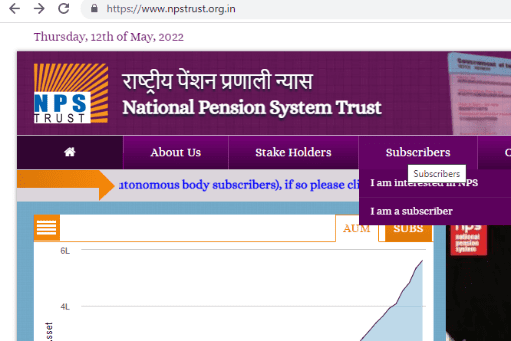Step 3: Click on the ‘Calculate the Pension Need’ option to reach the NPS calculator.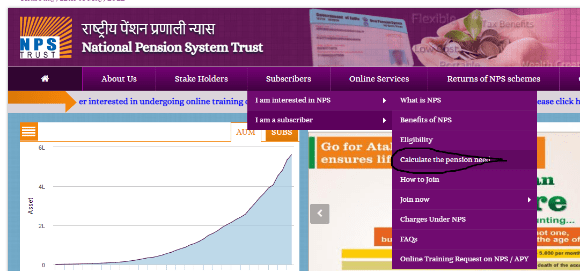Step 4: The next page will show the NPS calculator with standard calculations. Input your details for your calculation.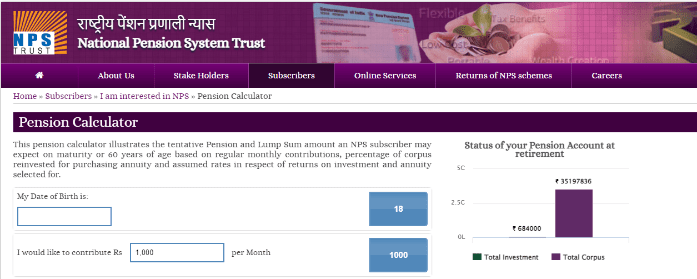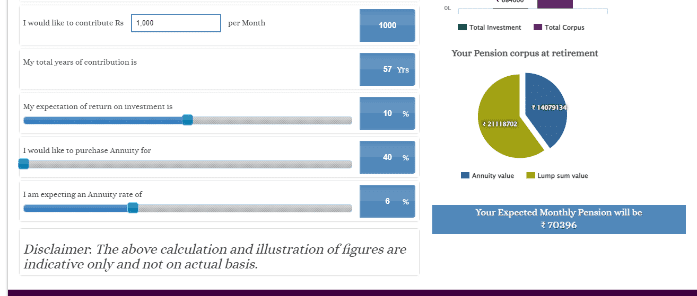Step 5: At this stage, provide the following details for the NPS calculator to calculate your pension savings:

• Date of birth: Based on this, the NPS calculator will reflect the number of years you will be able to contribute to the NPS.
• Investment amount: Enter your monthly contribution towards the NPS scheme.
• Expected return on investment: Choose the expected return on investment.
• Percentage of annuity to be purchased: The percentage of an annuity cannot be less than 40%.
• Expected annuity rate: Enter the expected rate of the annuity.

Once all the details are entered, the NPS calculator would show the details of your NPS account value at maturity.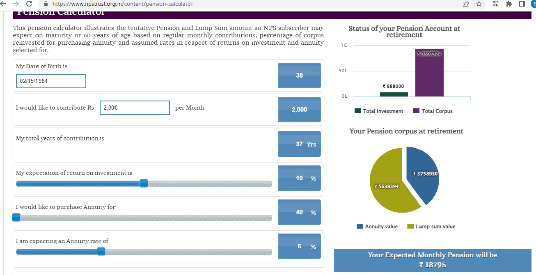## How the NPS calculator computes your monthly pension?

Let us assume that Sunaina, 38, is a private sector worker. She has decided to invest in the NPS scheme now and plans to contribute Rs 2,000 monthly till she is 60. She expects a return on investment of 9% annually. She is buying an annuity for 40% and expects a 7% rate of return on the annuity.

Total years of contribution: 37

Total investment: Rs 8,88,000

Total corpus generated: Rs 71,44,746

Lump-sum value: Rs 42,86,848

Annuity value: Rs 28,57,898

Expected monthly pension: Rs 14,289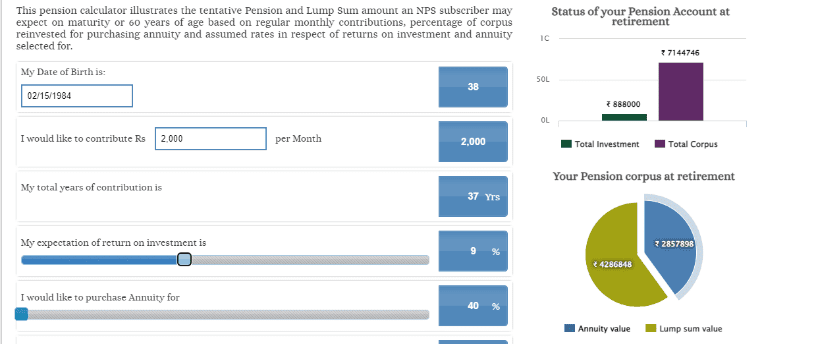Source:-https://housing.com/news/nps-calculator/

,

,

,

,

,

,